﻿ ArcFourManaged Members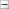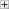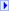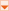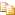The ArcFourManaged type exposes the following members.

#Constructors

NameDescription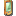ArcFourManaged
Initializes a new instance of ArcFourManaged class.

#Methods

NameDescriptionClear
Releases all resources used by the SymmetricAlgorithm class.
(Inherited from SymmetricAlgorithm.)CreateDecryptor()()()()
Creates a symmetric decryptor object with the current Key property and initialization vector (IV).
(Inherited from SymmetricAlgorithm.)CreateDecryptor(array<Byte>[]()[][], array<Byte>[]()[][])
Creates a symmetric ArcFour decryptor object with the specified Key and initialization vector (IV).
(Overrides SymmetricAlgorithm..::..CreateDecryptor(array<Byte>[]()[][], array<Byte>[]()[][]).)CreateEncryptor()()()()
Creates a symmetric encryptor object with the current Key property and initialization vector (IV).
(Inherited from SymmetricAlgorithm.)CreateEncryptor(array<Byte>[]()[][], array<Byte>[]()[][])
Creates a symmetric ArcFour encryptor object with the specified Key and initialization vector (IV).
(Overrides SymmetricAlgorithm..::..CreateEncryptor(array<Byte>[]()[][], array<Byte>[]()[][]).)Dispose()()()() (Inherited from SymmetricAlgorithm.)Dispose(Boolean)
Releases the unmanaged resources used by the SymmetricAlgorithm and optionally releases the managed resources.
(Inherited from SymmetricAlgorithm.)Equals
Determines whether the specified Object is equal to the current Object.
(Inherited from Object.)Finalize
Allows an Object to attempt to free resources and perform other cleanup operations before the Object is reclaimed by garbage collection.
(Inherited from Object.)GenerateIV
Generates a random initialization vector (IV) to be used for the algorithm.
(Inherited from ArcFour.)GenerateKey
Generates a random key to be used for the algorithm.
(Inherited from ArcFour.)GetHashCode
Serves as a hash function for a particular type. GetHashCode()()()() is suitable for use in hashing algorithms and data structures like a hash table.
(Inherited from Object.)GetType
Gets the Type of the current instance.
(Inherited from Object.)MemberwiseClone
Creates a shallow copy of the current Object.
(Inherited from Object.)ToString
Returns a String that represents the current Object.
(Inherited from Object.)ValidKeySize
Determines whether the specified key size is valid for the current algorithm.
(Inherited from SymmetricAlgorithm.)

#Fields

NameDescriptionBlockSizeValue
Represents the block size, in bits, of the cryptographic operation.
(Inherited from SymmetricAlgorithm.)FeedbackSizeValue
Represents the feedback size, in bits, of the cryptographic operation.
(Inherited from SymmetricAlgorithm.)IVValue
Represents the initialization vector (IV) for the symmetric algorithm.
(Inherited from SymmetricAlgorithm.)KeySizeValue
Represents the size, in bits, of the secret key used by the symmetric algorithm.
(Inherited from SymmetricAlgorithm.)KeyValue
Represents the secret key for the symmetric algorithm.
(Inherited from SymmetricAlgorithm.)LegalBlockSizesValue
Specifies the block sizes that are supported by the symmetric algorithm.
(Inherited from SymmetricAlgorithm.)LegalKeySizesValue
Specifies the key sizes that are supported by the symmetric algorithm.
(Inherited from SymmetricAlgorithm.)ModeValue
Represents the cipher mode used in the symmetric algorithm.
(Inherited from SymmetricAlgorithm.)PaddingValue
Represents the padding mode used in the symmetric algorithm.
(Inherited from SymmetricAlgorithm.)

#Properties

NameDescriptionBlockSize
Gets or sets the block size of the cryptographic operation in bits.
(Inherited from ArcFour.)FeedbackSize
Gets or sets the feedback size, in bits, of the cryptographic operation.
(Inherited from SymmetricAlgorithm.)IV
Gets or sets the initialization vector (IV) for the symmetric algorithm.
(Inherited from ArcFour.)Key
Gets or sets the secret key for the symmetric algorithm.
(Inherited from SymmetricAlgorithm.)KeySize
Gets or sets the size, in bits, of the secret key used by the symmetric algorithm.
(Inherited from SymmetricAlgorithm.)LegalBlockSizes
Gets the block sizes that are supported by the symmetric algorithm.
(Inherited from SymmetricAlgorithm.)LegalKeySizes
Gets the key sizes that are supported by the symmetric algorithm.
(Inherited from SymmetricAlgorithm.)Mode
Gets or sets the mode for operation of the symmetric algorithm.
(Inherited from ArcFour.)Padding
Gets or sets the padding mode used in the symmetric algorithm.
(Inherited from ArcFour.)